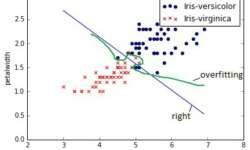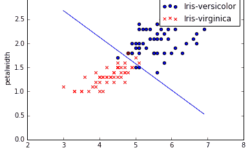## Regularized Logistic regressionPreviously we have tried logistic regression without regularization and with simple training data set. Bu as we all know, things in real life aren’t as simple as we would want to. There are many types of data available the need to be classified. Number of features can grow up hundreds and thousands while number of instances may be limited. Also in many times we might need to classify in more than two classes. The first problem that might rise due to large number of features is over-fitting. This is when learned hypothesis hΘ (x) fit training data too well (cost J(Θ) ≈ 0), but it fails when classifying new data samples. In other words, model tries to distinct each training example correctly by drawing very complicated decision boundary between training data points. As you can see in image above, over-fitting would be green decision boundary. So how to deal with over-fitting problem? There might be several approaches: Reducing number of features; Selecting different classification model; Use regularization. We leave first two out of question, because selecting optimal number of features is a different topic of optimization. Also we are sticking with logistic regression model for now, so changing classifier is…

## Implementing logistic regression learner with pythonLogistic regression is the next step from linear regression. The most real-life data have a non-linear relationship, thus applying linear models might be ineffective. Logistic regression is capable of handling non-linear effects in prediction tasks. You can think of lots of different scenarios where logistic regression could be applied. There can be financial, demographic, health, weather and other data where the model could be implemented and used to predict next events on future data. For instance, you can classify emails into spam and non-spam, transactions being fraud or not, tumors being malignant or benign. In order to understand logistic regression, let’s cover some basics, do a simple classification on data set with two features and then test it on real-life data with multiple features.